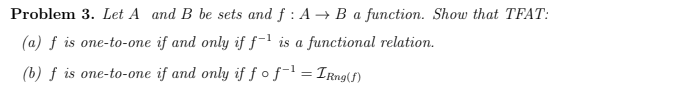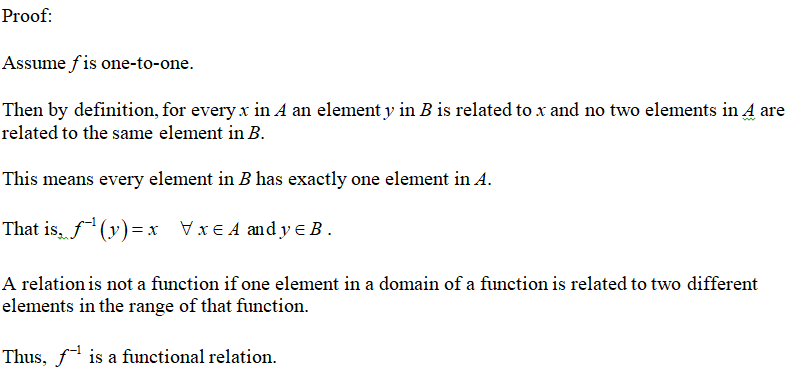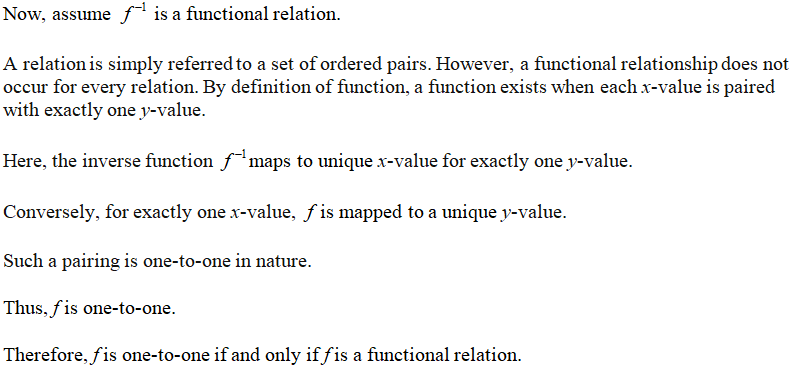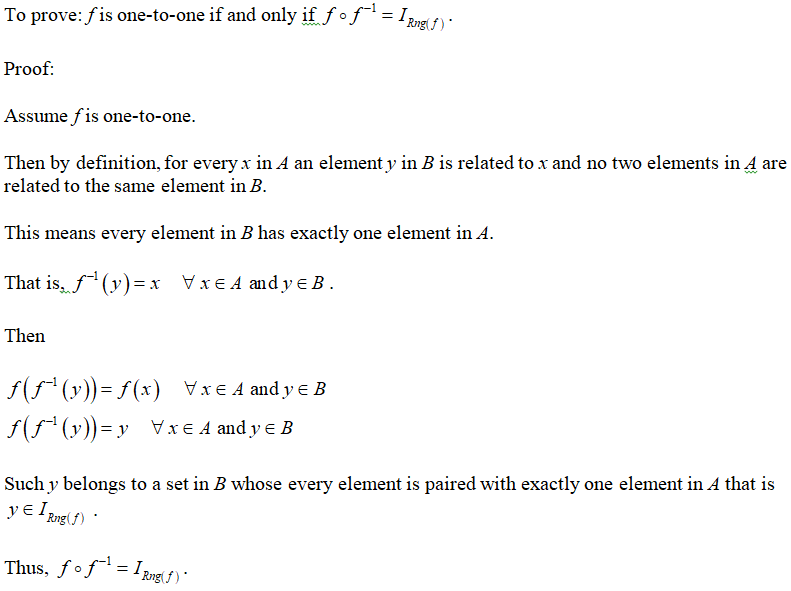# Problem 3. Let A and B be sets and f : A → B a function. Show that(a) f is one-to-one if and only if ƒ-' is a functional relation.(b) f is one-to-one if and only if fofl = IRng(f)TFAT:%3D

Question
1 views

Please solve this problem for me. TFAT means "the following are true".help_outlineImage TranscriptioncloseProblem 3. Let A and B be sets and f : A → B a function. Show that (a) f is one-to-one if and only if ƒ-' is a functional relation. (b) f is one-to-one if and only if fofl = IRng(f) TFAT: %3D fullscreen
check_circle

Proof of (a)Converse:Prroof of (b)...

### Want to see the full answer?

See Solution

#### Want to see this answer and more?

Solutions are written by subject experts who are available 24/7. Questions are typically answered within 1 hour.*

See Solution
*Response times may vary by subject and question.
Tagged in

### Math